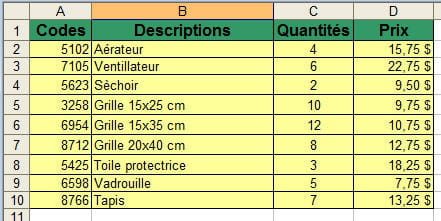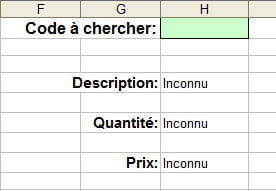0
Thanks

A few words of thanks would be greatly appreciated.

How To Use the VLOOKUP Function on ExcelThe VLOOKUP function on Microsoft Excel allows you to search a value in the first column of data and return the contents of another column.

Microsoft Excel: VLOOKUP Function

The basic syntax of the VLOOKUP function looks like this:
VLookup(value, table_array,index_number,matching_preferences)
.

For this tutorial, we will use the data in the following charts. Column A shows you Codes; Column B shows the Code Descriptions, Column C the Quantities, and Column D the Price:Using VLOOKUP (Excel 2003 and Excel 2007)

References

You must select the references in your table. In this example your reference is \$A\$2:\$D\$10. Once you made your selection click Insert > Name > Define=
• Excel 2007: The definition of the name is done via the Formula menu.

Target cells- where should data be generated?

In this example:
H1 = search term
H4 = Description found (column2 of our table)
H6 = Amount found (column 3 of our table)
H8 = Price found (column 4 of our table)

Formulas

The basic syntax of the VLOOKUP function is:
=VLOOKUP(value_search,table_array,no_index_col,range_lookup)
• H4
• =VLOOKUP(\$H\$1,CHAMPS,2,FALSE)
• To avoid message #N/A if nothing is found, use the following formula and "Unknown" will appear in the cell.
• =IF(ISNA(VLOOKUP(\$H\$1,CHAMPS,2,FALSE)),"Unknown",VLOOKUP(\$H\$1,CHAMPS,2,FALSE))
• Excel 2007 the formula can be shortened:

=IFERROR(VLOOKUP(\$H\$1,CHAMPS,2,FALSE),"Unknown")
• H6
• =VLOOKUP(\$H\$1,CHAMPS,3,FALSE)
• To avoid message #N/A if nothing is found, use the following formula and "Unknown" will appear in the cell.
• =IF(ISNA(VLOOKUP(\$H\$1,CHAMPS,3,FALSE)),"Unknown",VLOOKUP(\$H\$1,CHAMPS,3,FALSE))
• H8
• =VLOOKUP(\$H\$1,CHAMPS,4,FALSE)
• To avoid message #N/A if nothing is found, use the following formula and "Unknown" will appear in the cell.
• =IF(ISNA(VLOOKUP(\$H\$1,CHAMPS,4,FALSE)),"Unknown",VLOOKUP(\$H\$1,CHAMPS,4,FALSE))
• Excel 2007 the formula can be shortened:

=IFERROR(VLOOKUP(\$H\$1,CHAMPS,2,FALSE),"Unknown")

Note: In the formula put the parameter as FALSE if you want to find the exact value (#N/A if not found), and set it to TRUE if you want the value less than or equal to your search, and in this case the list of values ??must be sorted.
0
Thanks

A few words of thanks would be greatly appreciated.CCM is a leading international tech website. Our content is written in collaboration with IT experts, under the direction of Jeff Pillou, founder of CCM.net. CCM reaches more than 50 million unique visitors per month and is available in 11 languages.